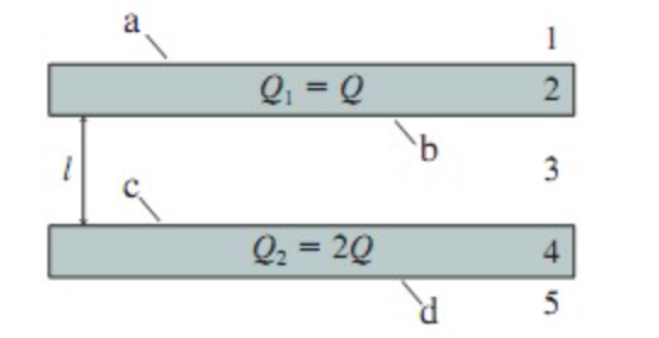###### FREE Expert Solution

In this problem, we'll determine electric fields using gauss law and use the electric fields to determine surface charge density.

Surface charge density:

$\overline{){\mathbf{\eta }}{\mathbf{=}}\frac{\mathbf{E}}{\mathbf{A}}}$

Gauss law:###### Problem Details

(Figure 1) shows two very large slabs of metal that are parallel and distance l apart. The top and bottom surface of each slab has surface area A. The thickness of each slab is so small in comparison to its lateral dimensions that the surface area around the sides is negligible. Metal 1 has total charge Q1=Q and metal 2 has total charge Q2=2Q.A.) Determine the electric field strength E1 in region 1. Give your answer as a multiple of Q/ε0.

B.) Determine the electric field strength E2 in region 2. Give your answer as a multiple of Q/ε0.
C.) Determine the electric field strength E3 in region 3. Give your answer as a multiple of Q/ε0.
D.) Determine the electric field strength E3 in region 3. Give your answer as a multiple of Q/ε0
C.) Determine the electric field strength E4 in region 4. Give your answer as a multiple of Q/ε0.
E.) Determine the electric field strength E5 in region 5. Give your answer as a multiple of Q/ε0.
F.) Determine the surface charge density ηa on the surface a. Give your answer as a multiple of Q/A.
G.) Determine the surface charge density ηb on the surface b. Give your answer as a multiple of Q/A.
H.) Determine the surface charge density ηc on the surface c. Give your answer as a multiple of Q/A.
I.) Determine the surface charge density ηd on the surface d. Give your answer as a multiple of Q/A.